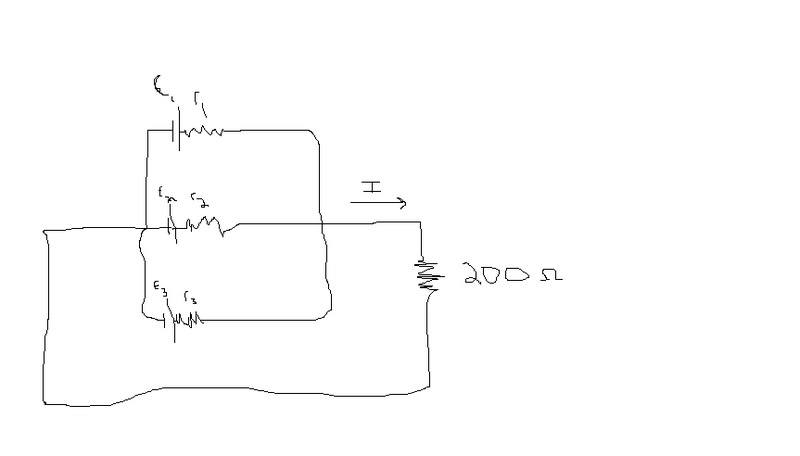# Battery Problem (finding current w/ batteries in parallel)

• aleksxxx

## Homework Statement

Find the current flowing through the 200ohm resistorE1=5v
E2=10v
E3=15v
r1=3ohms
r2=6ohms
r3=9ohms

## The Attempt at a Solution

I know that I1+I2+I3=I
I can't seem to get a loop rule that will allow me to solve for I though.

I came up with the equivalent resistance in the three resistors equal to 1.6ohms.

I tried to draw a simplified circuit diagram, but i am not sure how to do that with different voltages on batteries in parallel.

thanks

Equivalent resistance won't help. Youve got 4 unknowns and need 4 equations. Theres enough information to use one junction equation and 3 loops. Make use of the inner loops, and use a matrix to avoid algebra mistakes whenever possible

Equivalent resistance won't help. Youve got 4 unknowns and need 4 equations. Theres enough information to use one junction equation and 3 loops. Make use of the inner loops, and use a matrix to avoid algebra mistakes whenever possible

i tried that and i seem to just keep proving that I2=I2, I1=I1, etc...

http://www.bluebit.gr/matrix-calculator/linear_equations.aspx

Plug your equations into this matrix calculator to see if youre missing a necessary loop or if youre just hung up on how to reach the solution

I worked on the loop equations more and found I1, I2, and I3 in terms of I.

I then substituted those into the I1+I2+I3=I equation getting a current (I) of .041 A leading to a deltaV of 8.1V.

Does this sound right? The Current was .137A when connected in series and the deltaV of the 200 ohm resistor was 27.4V.

Thanks